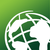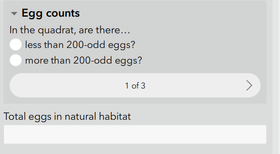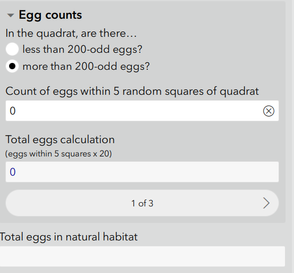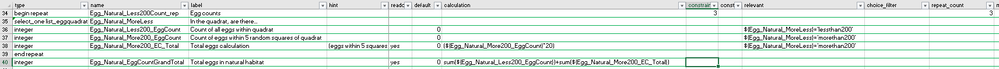# Calculation only works when both choices selected

264
2
02-15-2022 01:15 PMNew Contributor III

I have a repeat with a select_one question inside it.The select_one opens either an integer field or a simple calculation:or:I want the Total eggs in natural habitat field to be the sum of Count of all eggs within quadrat and Total eggs calculation. But it doesn't populate if every choice from the select one is the same, only if a mixture of choices is made. Is there a way to make the calculation work if all choices are the same?

I have attached the excel form if the image is not clear.Many thanks

Tags (3)
1 Solution

Accepted SolutionsbyEsri Regular Contributor

The total eggs calculation is not working when only one of the choices is selected for all repeats because one half of the sum is null (i.e. if one of the eggcount questions remains not relevant across all repeats, its value is null).

One way around this would be to use the coalesce() function in the total calculation, which will overcome any null values (replacing null with zero). Something like:

coalesce(sum(\${Egg_Natural_Less200_EggCount}), 0) + coalesce(sum(\${Egg_Natural_More200_EC_Total}), 0)

Hope this helps. Best, Jim

2 RepliesbyEsri Regular Contributor

The total eggs calculation is not working when only one of the choices is selected for all repeats because one half of the sum is null (i.e. if one of the eggcount questions remains not relevant across all repeats, its value is null).

One way around this would be to use the coalesce() function in the total calculation, which will overcome any null values (replacing null with zero). Something like:

coalesce(sum(\${Egg_Natural_Less200_EggCount}), 0) + coalesce(sum(\${Egg_Natural_More200_EC_Total}), 0)

Hope this helps. Best, JimNew Contributor III

Perfect solution, many thanks Jim!